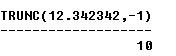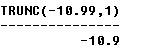## SQL TRUNC (Number) Function

SQL TRUNC (number) function is used to round numeric values. SQL TRUNC function is similar to CEIL and FLOOR functions except that in this you can mention the degree of precision you want to round up to. The syntax is:

TRUNC (number, round_to);

Second parameter (round_to) defines the degree of precision you want in rounding this number.

ROUND vs TRUNC:
SQL TRUNC function differs from ROUND in that it just removes the number of digits mentioned in second parameter. There is no rule for rounding to next whole number. If the value is 2, it will remove all digits after 2 decimal places; if value is -1, it will remove all digits to the right of decimal place and enter zero to 1st place to left of decimal. If you don’t pass any value for second parameter, it will remove all digits left to decimal place.

Examples:
The following statements illustrate the use of SQL TRUNC function on couple different numbers:

SELECT TRUNC (2.32234234, 2) FROM DUAL;

OUTPUT:SELECT TRUNC (12.342342, -1 ) FROM DUAL;

OUTPUT:SELECT TRUNC (-5.45, -1) FROM DUAL;

OUTPUT:SELECT TRUNC (-10.99, 1) FROM DUAL;

OUTPUT: